# Standard Form

Standard Form

Julia asked her kids, "do you know how old the Earth is?"They thought and said that the Earth was older than her, them, and their grandparents. Julia told them that the Earth was believed to have been formed over 4.5 billion years ago. She asked them if they could try counting this number on their fingers. When they replied that they couldn't, she told them that a number as huge as this can be expressed mathematically in its standard form only.

In this chapter, we will explore the world of standard form. The journey will take us through standard form of fractions, standard form of equation, standard form formula, standard form of slope and standard form in algebra, a few tips, such as the thumb rule, and interesting ways to understand standard form in simpler way.

## Lesson Plan

 1 What is Standard Form? 2 Tips and Tricks 3 Important Notes on Standard Form 4 Solved Examples on Standard Form 5 Interactive Questions on Standard Form

## What is Standard Form?

A standard form is a form of writing a given mathematical concept like an equation, number, or an expression in a form that follows certain rules.

4.5 billion years is written as 4,500,000,000 years

As you can see here, writing a large number like 4.5 billion in its number form is not just ambiguous but also time-consuming and there are chances that we may write a few 0’s less or more while writing in the number form.

So, to represent very large or very small numbers concisely, we use the standard form.

## Standard Form Rules

The process of writing a given mathematical concept like an equation, number, or an expression in the standard form follows certain rules.

Depending upon which mathematical concept we are dealing with, the standard form formula will vary.

For example, the standard form of $$4,500,000,000 = 4.5 \times 10^9$$

What about the fractions $$\dfrac{14}{22} \text {and} \dfrac{13}{6}$$? Are these standard forms of fractions?

In the case of fractions, we need to ensure that in the standard form of fractions, the numerator and denominator must be co-prime.

That means they have no common factor other than 1

So, the standard form, also called as the simplest form of the fraction $$\dfrac{14}{22} \text {is} \dfrac{7}{11}$$

The fraction $$\dfrac{13}{6}$$ is already in its simplest form as 13 and 6 are co-prime.

What we have observed in the above cases are the standard forms of writing a decimal number and fractions.

## How do we write in Standard Form?

Standard form has different meanings depending on which country you are in.

In the United Kingdom and countries using UK conventions, the standard form is another name for scientific notation.

$$3890 = 3.89 \times 10^3$$

In the United States and countries using US conventions, the standard form is the usual way of writing numbers in decimal notation.

Standard form $$= 3890$$

Expanded form $$= 3000 + 800 + 90$$

Written form $$=$$ Three thousand eight hundred and ninety

We now proceed to study the standard form in algebra.

Standard form in algebra includes some major topics like polynomials, linear and quadratic equations.

Let’s begin with polynomials.

## Standard Form of a Polynomial

Consider the algebraic expressions:

\begin{align} x^3+7x^2&-8x \\ 4x^5+7x^4&-5x^2-10\\y^2+7y^6&-8y-y^9 \\z^4+7z^5&-8z^2-12z+45\end{align}

Can you identify the correlation between all these expressions?

All these belong to the family of Polynomials.

In the above examples, the first two are in the standard form whereas the last two are not.

The rules for writing a polynomial in standard form are very simple.

1. Write the terms in the descending order of their powers (also called as exponents).

2. Ensure the polynomial contains no like terms.

The standard form is:

 $$a_nx^n + a_{n-1}x^{n-1}.....+a_0$$

Thus, standard form of $$y^2+7y^6-8y-y^9 = -y^9+7y^6+y^2-8y$$

Standard form of

\begin{align}\!z^4\!+\!7z^5\!-\!8z^2\!-\!12z\!+\!45=\\7z^5\!+\!z^4\!-\!8z^2\!-\!12z\!+\!45\end{align}Tips and Tricks

The thumb rule: D-U for writing a polynomial in its standard form.

D stands for Descending and U stands for unlike terms.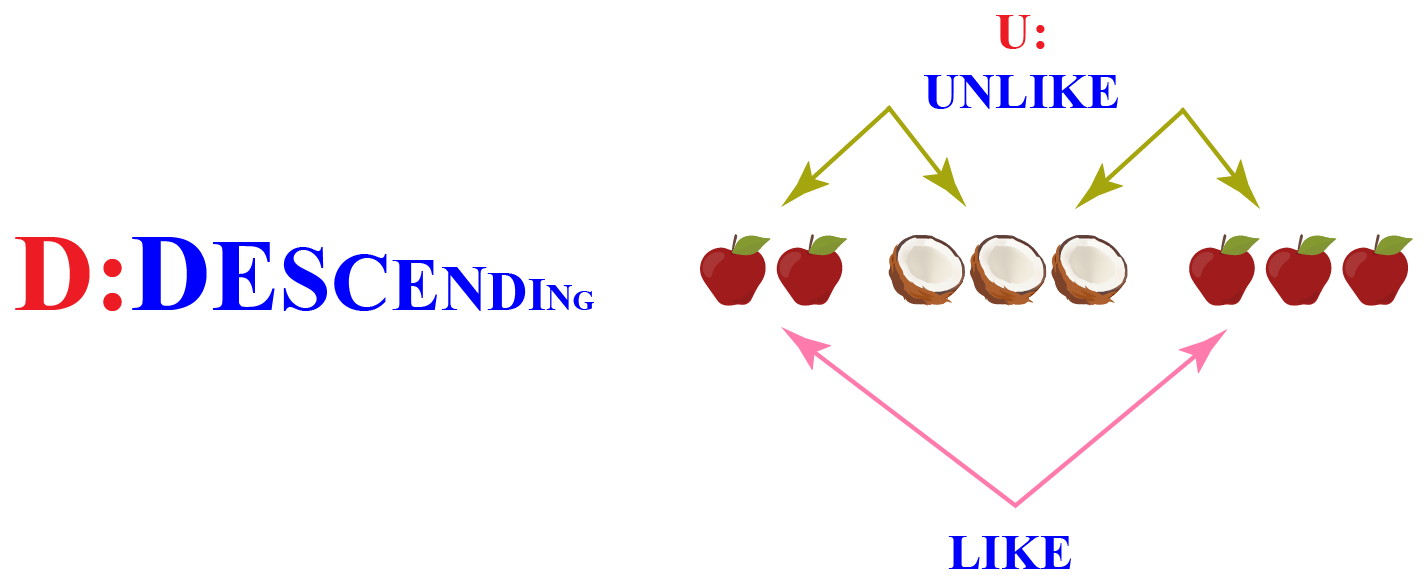## Standard Form of a Parabola

"What goes up must come down" - this is a well-known law of gravity.

How is it applicable to our topic?

Let's observe the shape of a banana and the motion of a dolphin in the water.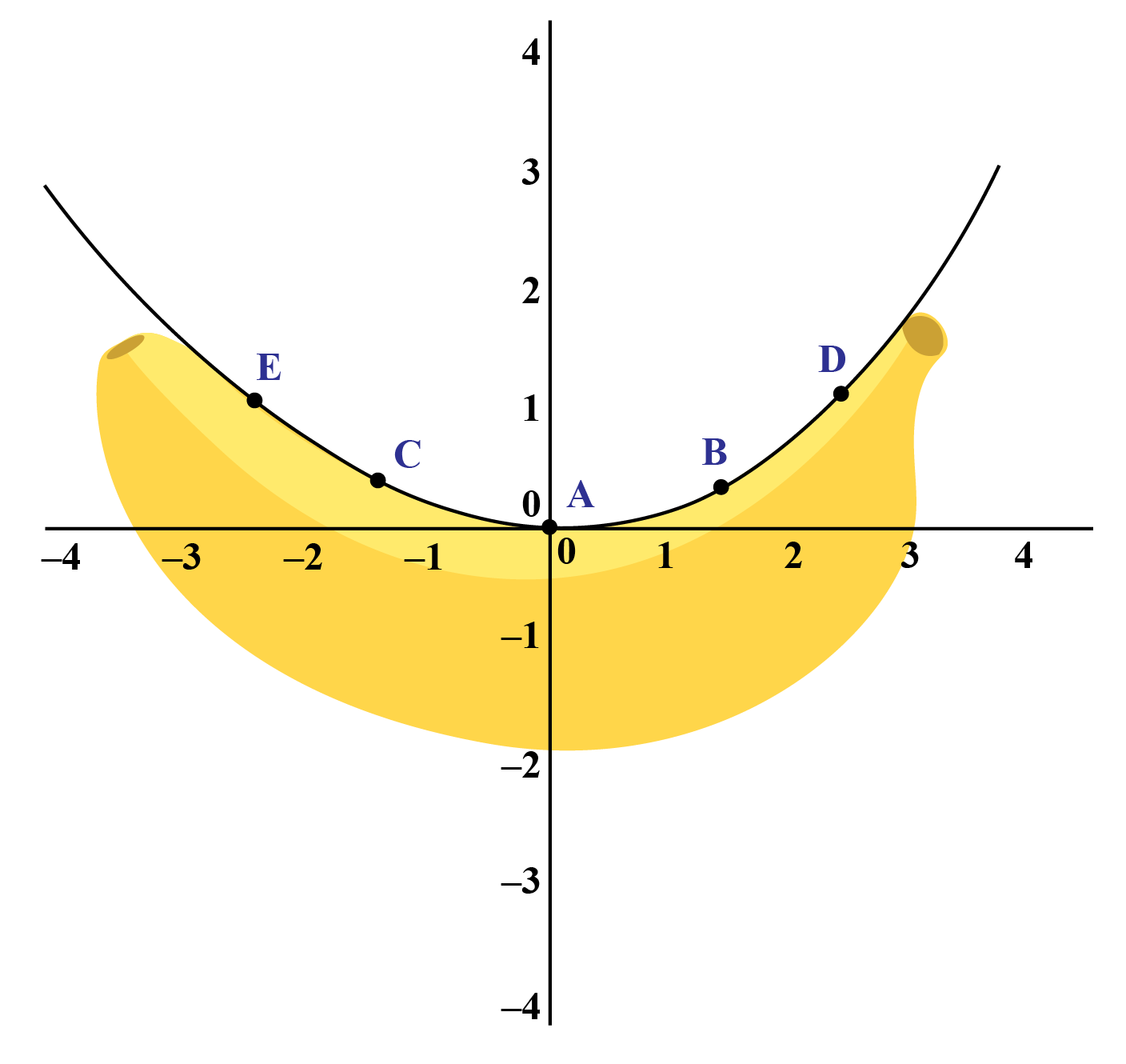Both of them form a simple curve shaped like an arch.

This is called a parabola in Mathematical terms.

A parabola is the graph of a quadratic function.

The standard form of parabola is:

 $$f (x) = ax^2 + bx + c$$

Note: It contains only one squared term.

The word parabola originated in the late 16th Century from para meaning "beside" and bolē meaning "a throw" (from the verb ballein).

The following graphs are two typical parabolas; their x and y-intercepts are marked by green dots, and the vertex of each parabola is marked by a blue dot.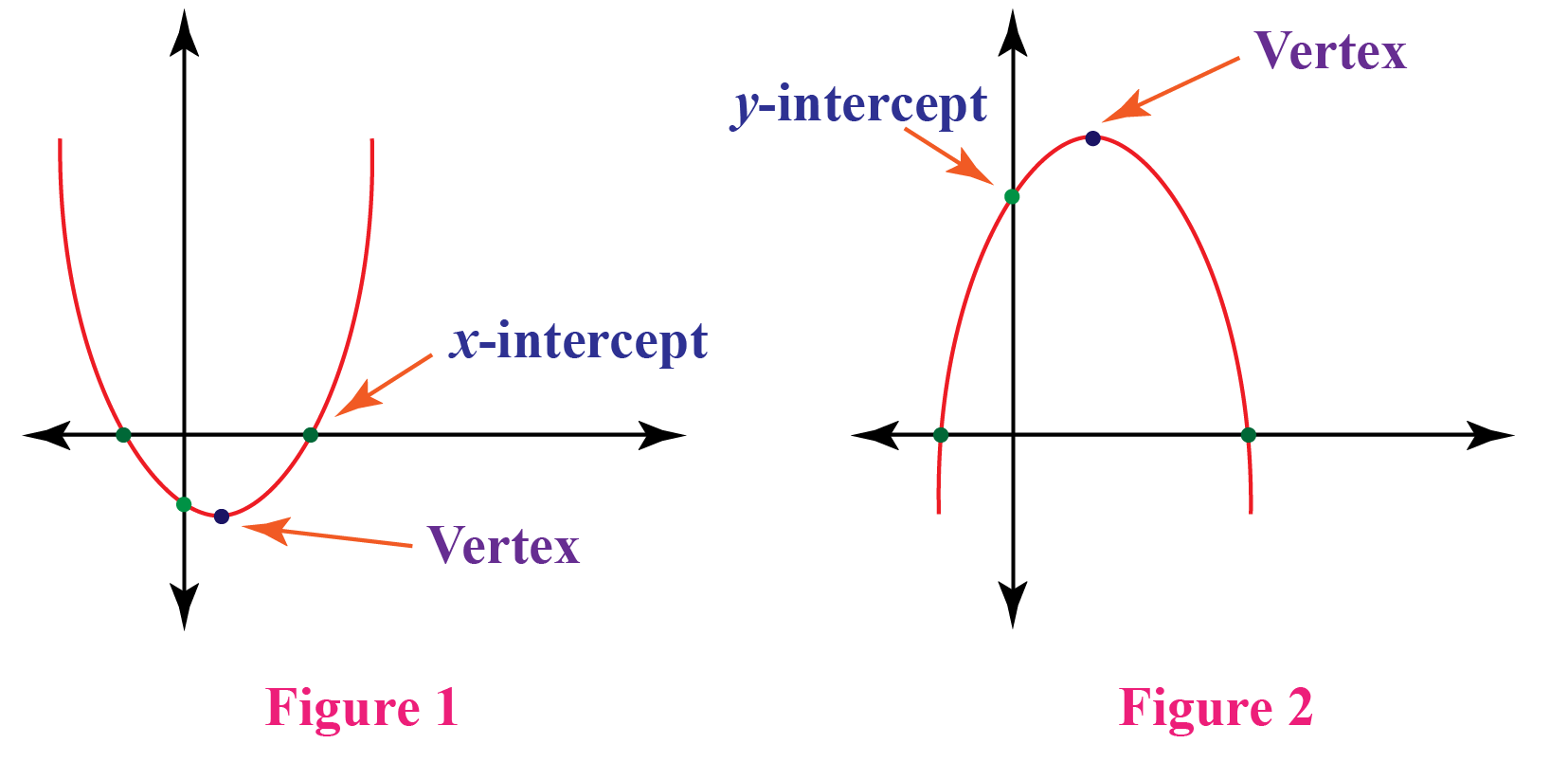For any quadratic polynomial $$ax^2+bx +c = y \text { where } a\neq 0$$, the graph will have one of the two shapes as shown above.

When a > 0, it represents the first parabola (figure 1) which opens upward (U-shaped).

When a < 0, we obtain a parabola that opens downward (inverted U-shaped) as shown in figure 2

Now when we put y = 0 in the above equation, we get the x-intercept which is also called as the roots of the equation: $$ax^2+bx +c = 0$$

Finding the roots provides us with the turning point of the parabola.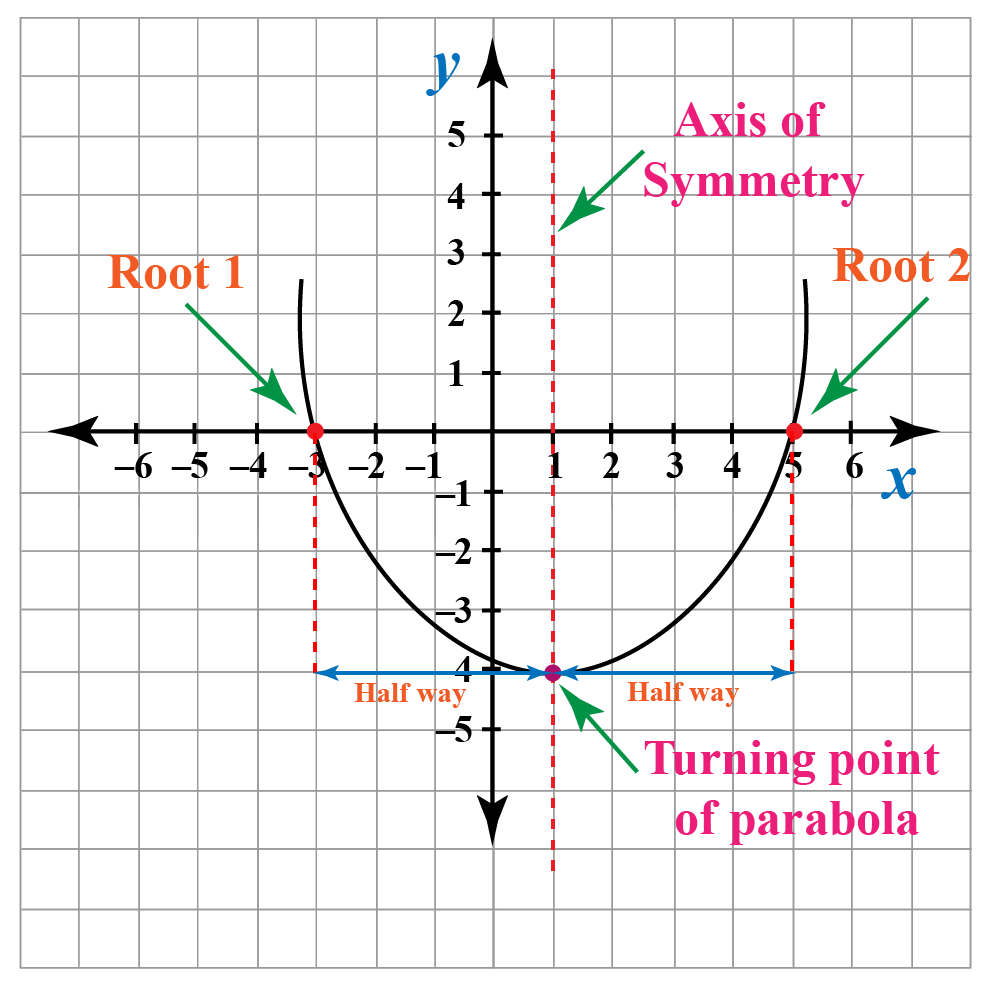The vertical line which is drawn from the turning point is called the axis of symmetry.

It is calculated using the formula:

 $$x = \dfrac{- b}{ 2a}$$

### Example:

For the equation $$x^2 + 3x-4=0$$, the equation of axis of symmetry is $$x = \dfrac{- b}{ 2a}= \dfrac{- 3}{ 2}$$.

## Standard Form of a Linear Equation

Look at the following images.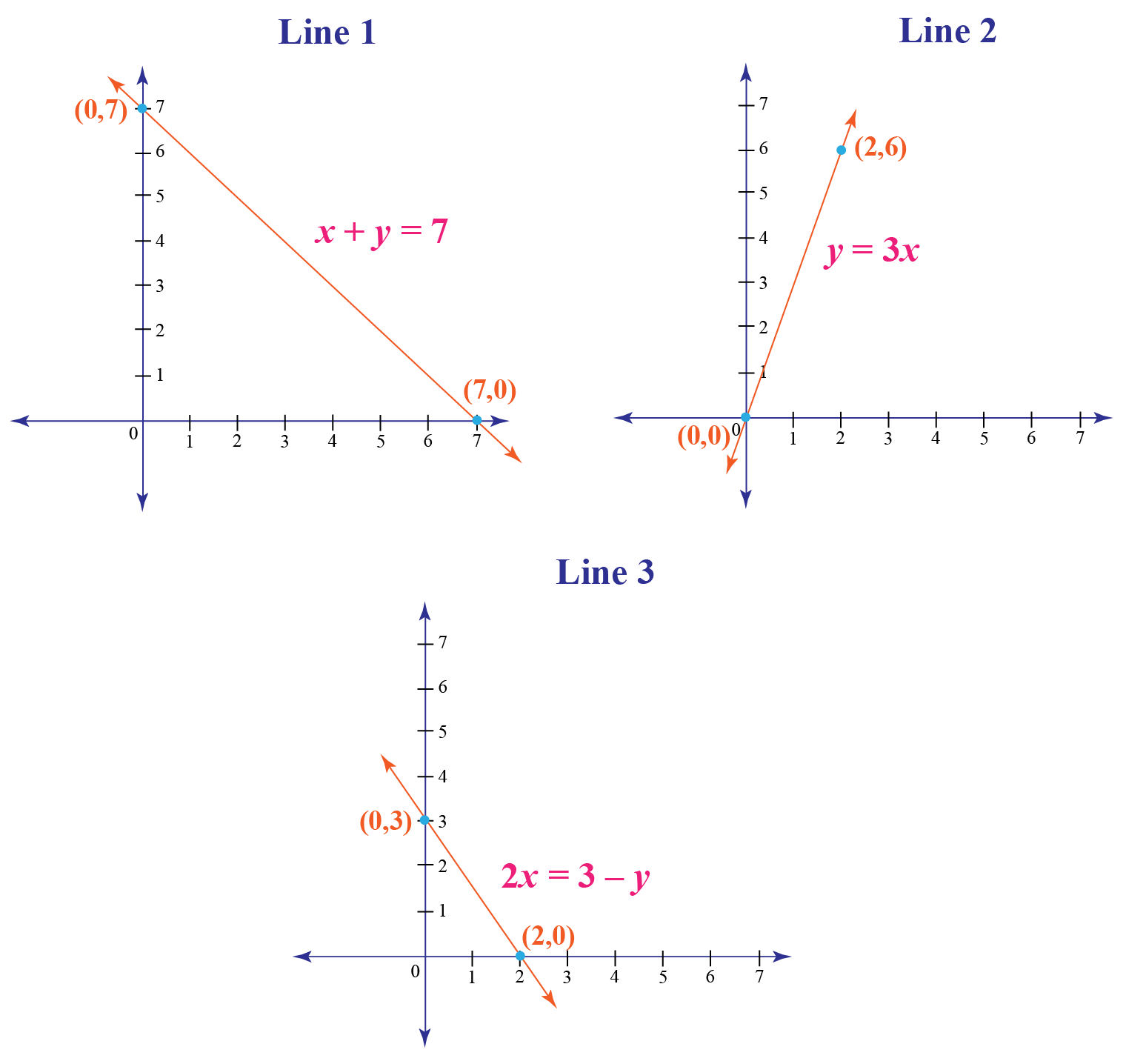All these are images of lines; observe the equations of each one of them.

Each of them has degree 1

Hence, they are called linear equations in two variables.

Can we write them in the form: $$Ax+ By = C?$$

Of course, we can!

Let’s see how.

Line 1: \begin{align} x\,+\,y\,&=\,7\\i.e. 1x+1y&=7\\Here, A= 1, B&=1 \,\text{and }\, C=7\end{align}

Line 2: \begin{align} \,y\,&=\,3x\\i.e. 3x-1y&=0\\Here, A= 3, B&=-1 \,\text{and } \,C=0\end{align}

Line 3: \begin{align} 2x\,&=\,3-y\\i.e. 2x+1y&=3\\Here, A= 2, B&=1 \,\text{and }\, C=3\end{align}

Therefore, what we see here is the standard form of equation which is linear.

 $$Ax+ By = C$$

## Standard Form of a Line

As discussed in the above section, a linear equation geometrically represents a line.

Hence, the standard form of a line is same as that of a linear equation.

i.e. $$Ax+By=C$$

### Slope:

We can also determine the slope of a given line.

If we come across an equation in standard form that we are required to present graphically, we must convert it to slope-intercept form.

To do this, we must solve the equation for y.

For slope, we write the equation in the form: $$y = mx+c$$

Here $$m$$ represents the slope of the line.

Example 1:

Consider $$5x+4y=10$$

Let us solve this equation.

\begin{align} 5x+4y&=10\\4y&=10-5x\\y&=\dfrac{-5x+10}{4}\\&=\dfrac{-5x}{4}-\dfrac{10}{4}\\&=\dfrac{-5}{4}x-\dfrac{5}{2}\end{align}

$$\therefore Slope= \dfrac{-5}{4}$$

Given two points in a plane, slope can be defined as the ratio of change in the value of y to the change in the value of $$x.$$

Standard form of slope is:

 $$m=\dfrac {y_2 – y_1}{x_2 – x_1}$$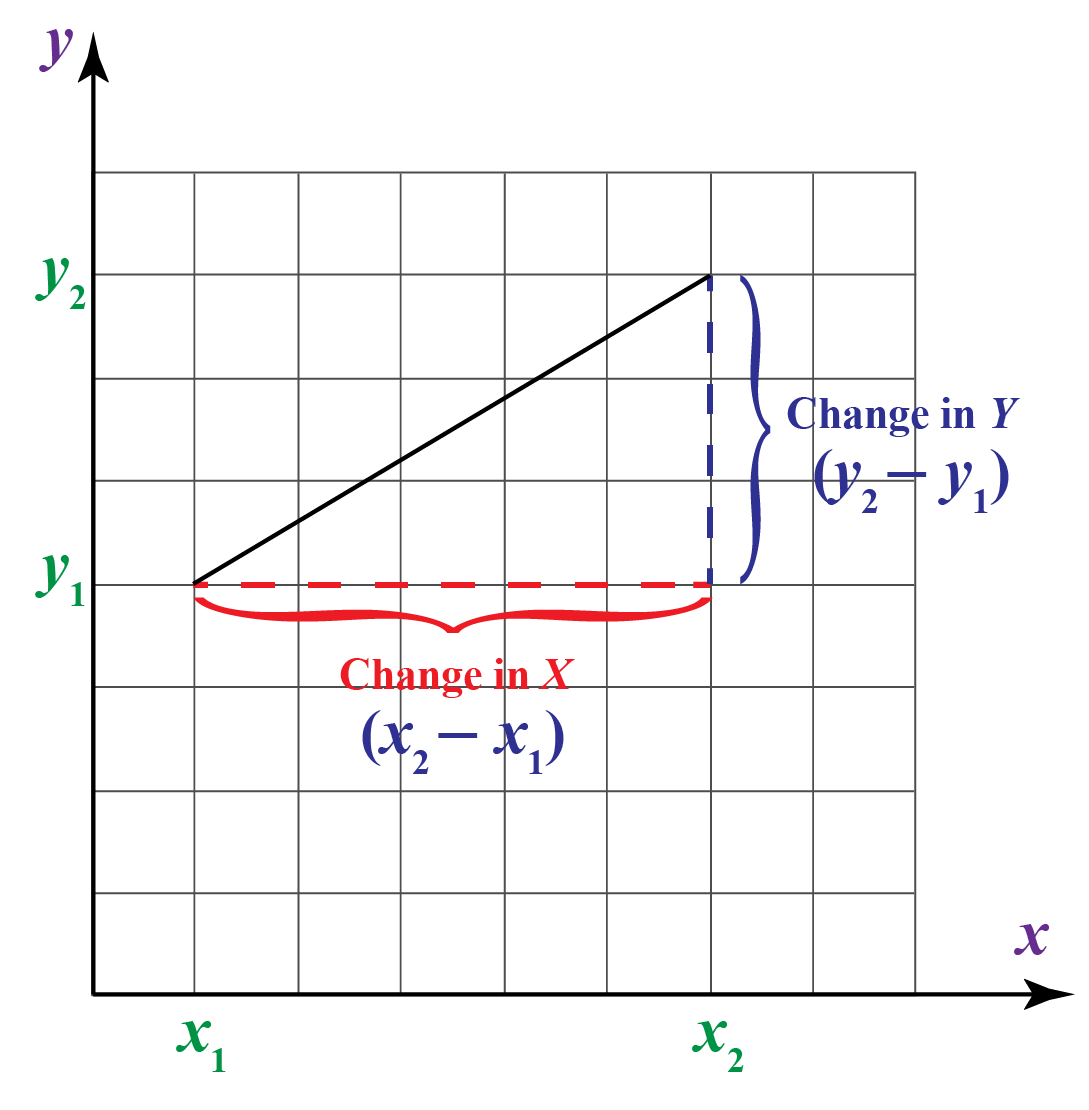Example 2:

Let's apply (4, 2) and (5, 6) into the slope formula.

We get slope $$= \dfrac {y_2 – y_1}{x_2 – x_1}=\dfrac {6 – 2}{5 – 4}= 4$$

Types of Slope:

Observe the figure below to understand the nature of slope.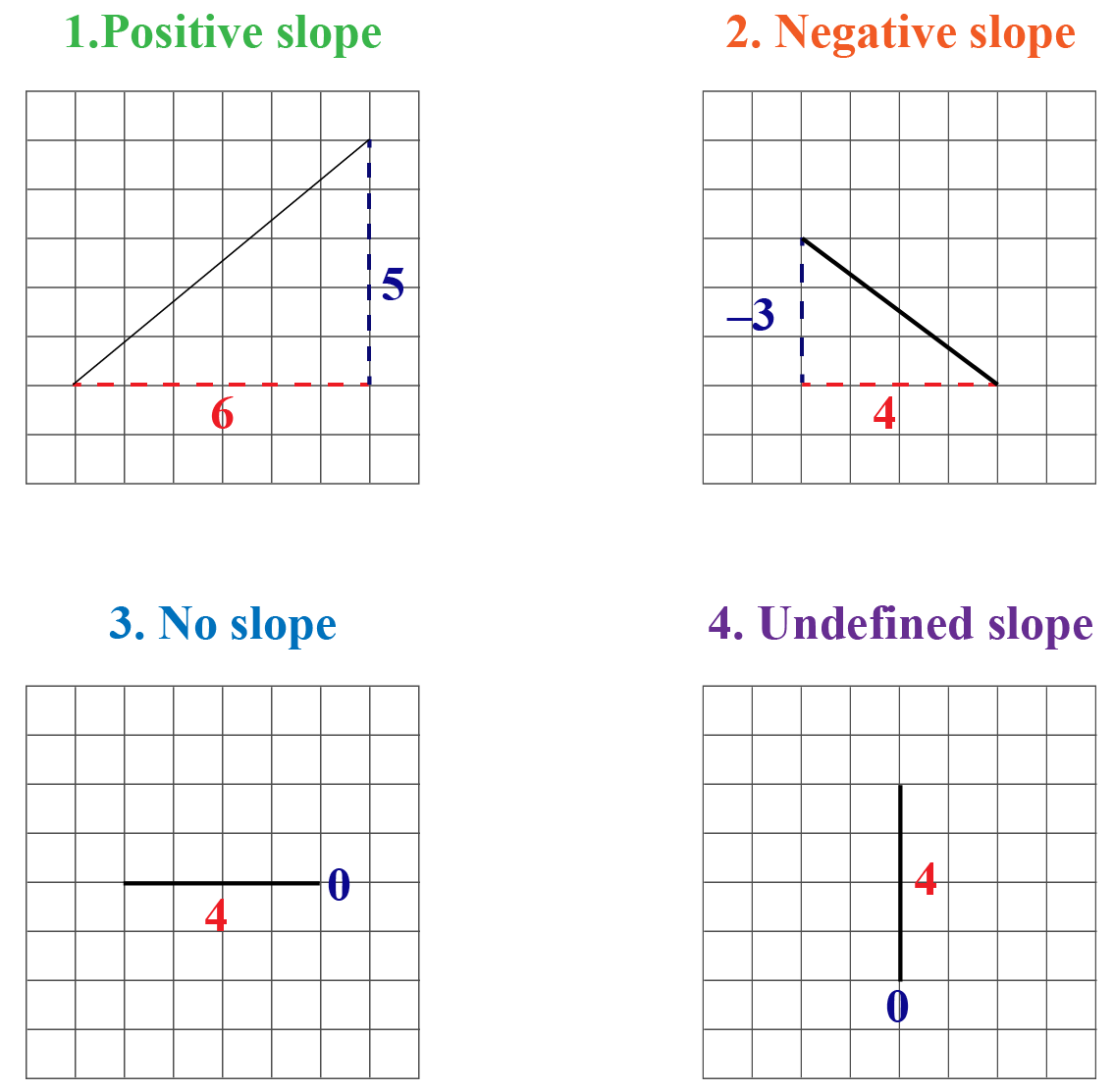You may calculate slope using the formula $$Slope = \dfrac{\text {Change in y}}{\text {Change in x}}$$.

In case 1, the value of slope is positive; in case 2, it is negative.

In case 3, $$Slope = \dfrac {0}{4}=0$$ and in case 4 $$Slope = \dfrac {4}{0}$$ which is not defined.

Take a look at the slope being formed by different lines in the simulation below.

## Solved Examples

 Example 1

Liza is trying to find out which of the following equations represent the given graph.

As there are no values of the coordinates given, she is not able to decide.

$$1. \,x^4+7x^2-5x =4\hspace{1cm} 2.\,4x+5y=0$$

$$3.\,y^2+7y^6-9y=y^3 \hspace{1cm} 4. \,3z^4+7z^5=-12z$$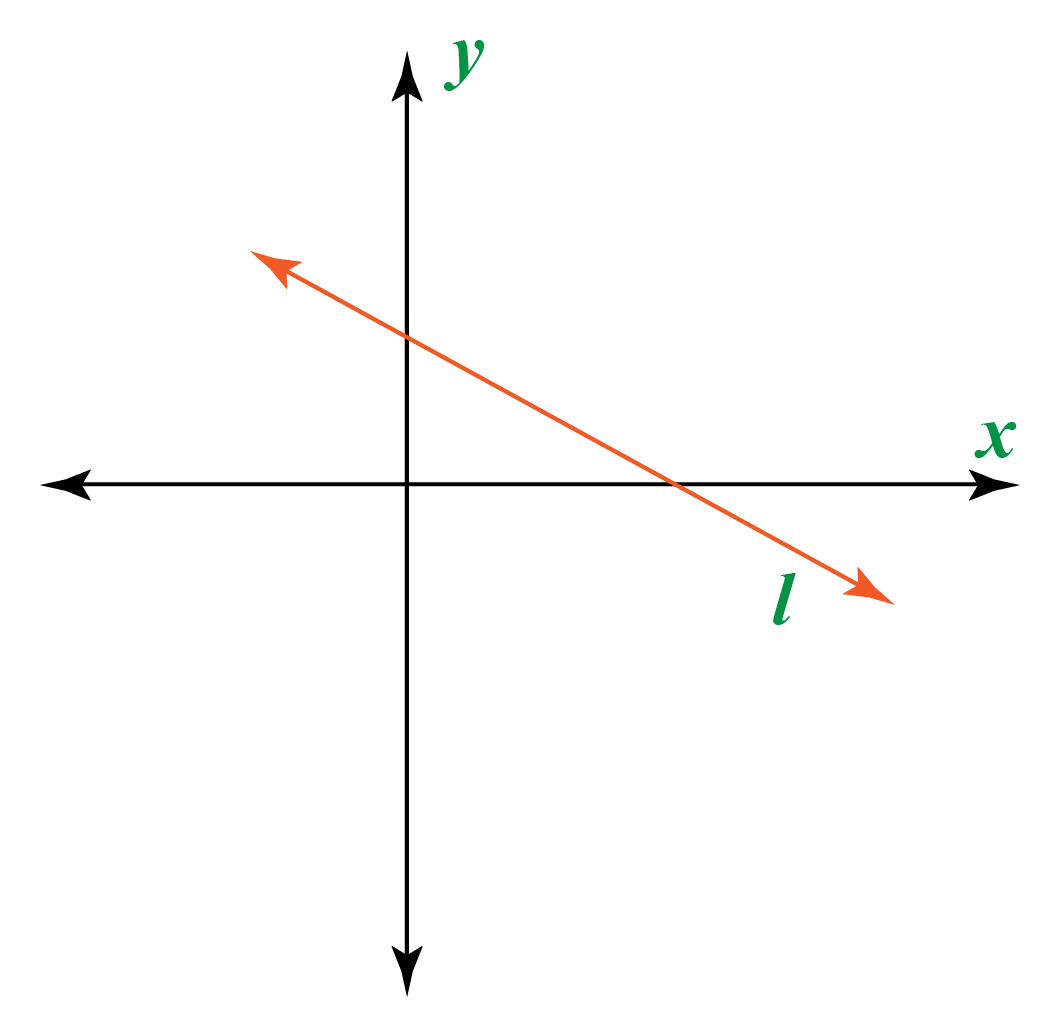How can we use standard form concept to solve her problem?

Solution

The graph given here represents a line.

Now, a line represents a linear equation in two variables which has a degree 1

Out of the four equations given above, only option (b) is linear.

 $$\therefore$$The graph represents the equation $$4x+5y =0$$
 Example 2

Anna showed her class notes on polynomials to her teacher.

The teacher returned her notes with a remark "Write the polynomial  $$x^2 -10x+16-x^2+x^5-3x^4 + 3x^2$$ in standard form."

What is the correct format Anna should have used?

Solution

To write a polynomial in standard form, two rules must be taken care of.

Rule : 1. Write the terms in descending order of their powers.

2. All terms must be unlike.

Let us first arrange in descending order.

$$x^2 - 10x +16 - x^2 +x^5 -3x^4 +3x^2 = x^5 - 3x^4 + x^2 -x^2 + 3x^2 - 10x +16$$

After adding like terms, we get

$$x^5- 3x^4+3x^2-10x+16$$

 $$\therefore \text{ Standard form is }x^5- 3x^4+3x^2-10x+16$$
 Example 3

James is looking at the parabola and trying to find the equation of axis of symmetry.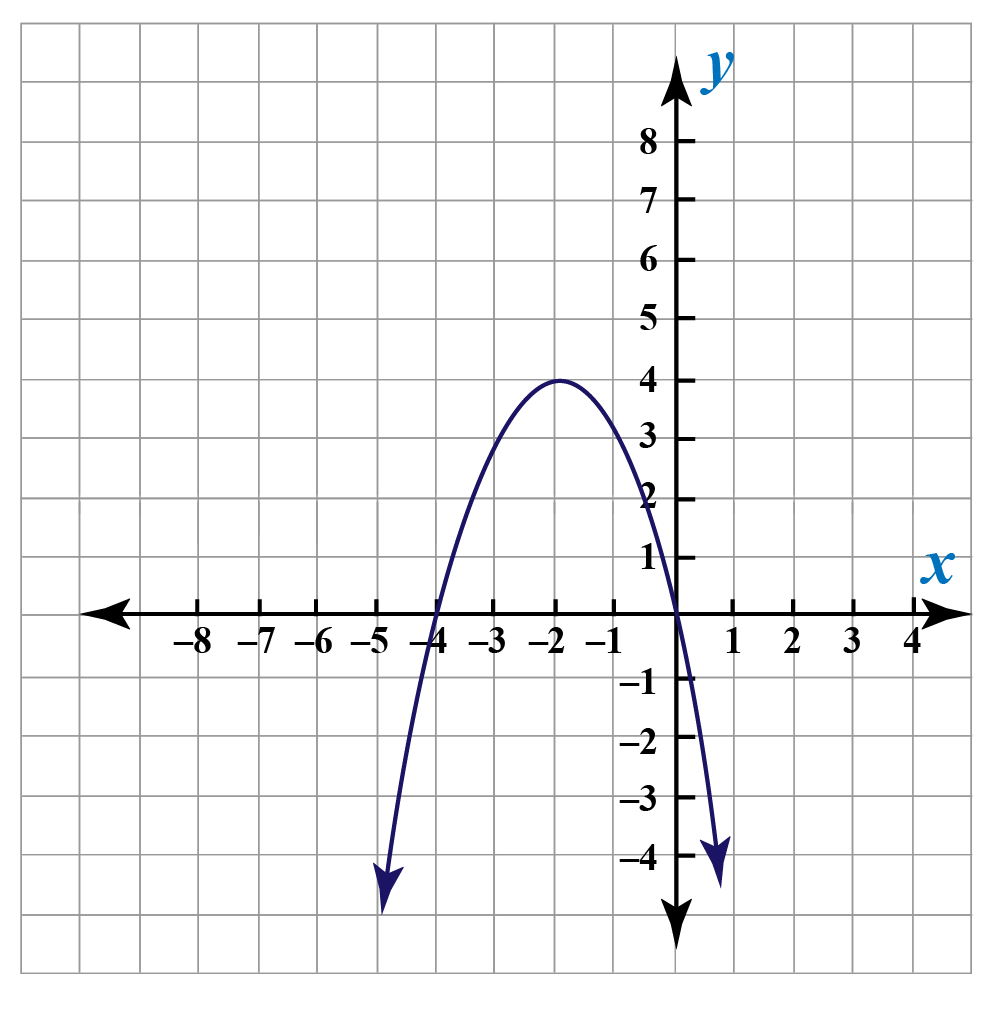How will he get the equation?

Solution

The x-coordinate of the vertex gives the equation of the axis of symmetry.

The vertex has coordinate $$(-2,4)$$

Its x-coordinate is $$-2$$

Thus, the equation of the axis of symmetry is $$x = -2$$

 $$\therefore \; x=-2$$ is the equation of axis of symmetry

## Interactive Questions

Here are a few activities for you to practice.

## Let's Summarize

We hope you enjoyed learning about standard form with the simulations and practice questions. Now you will be able to easily solve problems on standard form of fractions, standard form of equation, standard form formula, standard form of slope and standard form in algebra.

At Cuemath, our team of math experts is dedicated to making learning fun for our favorite readers, the students!

Through an interactive and engaging learning-teaching-learning approach, the teachers explore all angles of a topic.

Be it worksheets, online classes, doubt sessions, or any other form of relation, it’s the logical thinking and smart learning approach that we, at Cuemath, believe in.

## 1. How do you write in standard form?

We write numbers in standard form using the rules for respective mathematical concepts.

For example, a linear equation has a standard form $$Ax+By = C$$.

## 2. How does the standard form look like?

The standard form of a line is: $$Ax+By = C$$

For polynomials, the standard form is: $$a_nx^n + a_{n-1}x^{n-1}.....+a_0$$

## 3. What is the meaning of standard form?

A standard form is a form of writing a given mathematical concept like an equation, number or expression in a form that follows certain rules.

More Important Topics
Numbers
Algebra
Geometry
Measurement
Money
Data
Trigonometry
Calculus
More Important Topics
Numbers
Algebra
Geometry
Measurement
Money
Data
Trigonometry
Calculus